## Introduction

The prettyB changes the default values of some standard plotting functions. It is not a replacement for ggplot2 (or other plotting packages), instead, it’s meant to just make the base graphics a little bit nicer. Due to the underlying structure of base graphics & S3 objects, some things aren’t possible. In particular, changing how non-exported plot-methods are displayed.

The general idea is the argument signitures are exactly the same as base graphics. But there are just a bit prettier. The current methods that are available are

• `plot_p()`
• `barplot_p()`
• `boxplot_p()`
• `hist_p()`

## Histogram examples

The important point to note with this histogram method is the `breaks` argument now has a new default. Instead of `breaks = "Sturges"`, the default is `breaks = "fd"`. In general, this is a better default (but not always)

``````x = rlnorm(100)
hist(x)
hist_p(x)``````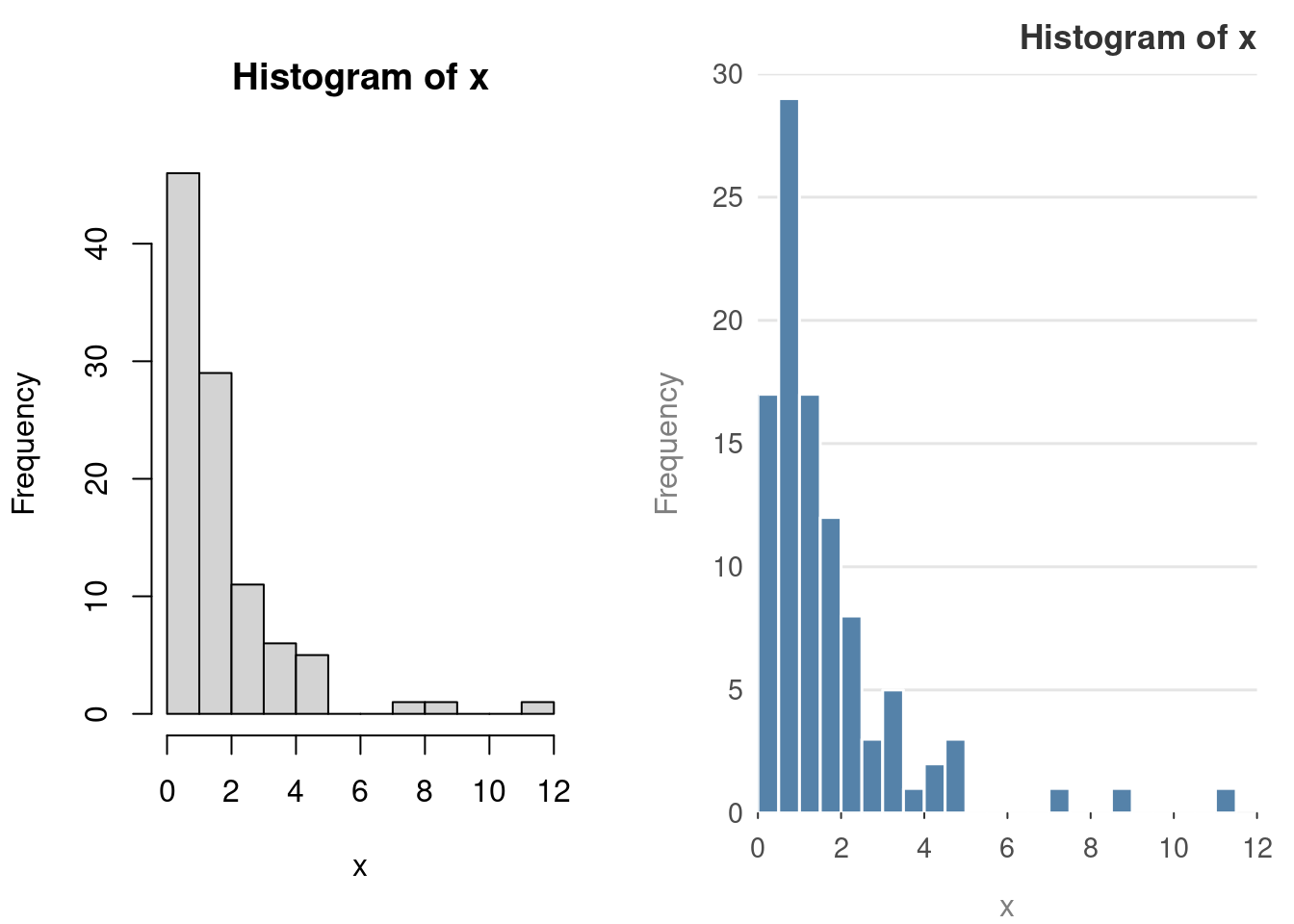Standard arguments work as well

``````y = rnorm(100)
hist(y, main = "Base Graphics", sub = "Sub heading",
xlab = "x-axis", ylab = "y-axis")
hist_p(y, main = "prettyB",  sub = "Sub heading", xlab = "x-axis", ylab = "y-axis")``````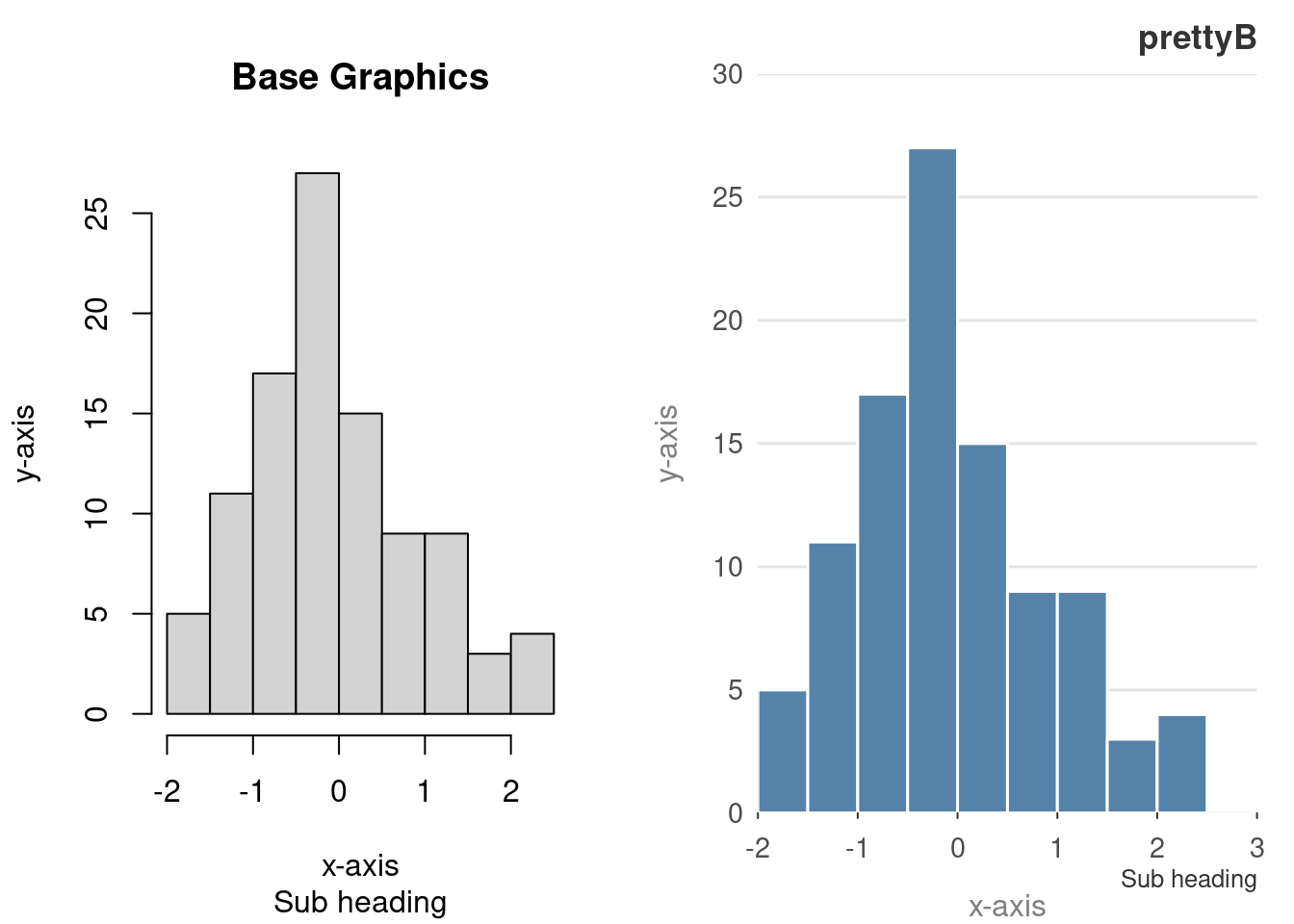Colours and frequencies also work as is expected

``````z = rt(100, 4)
hist(z, col = "grey60", freq = FALSE)
hist_p(z, col = "grey60", freq = FALSE)``````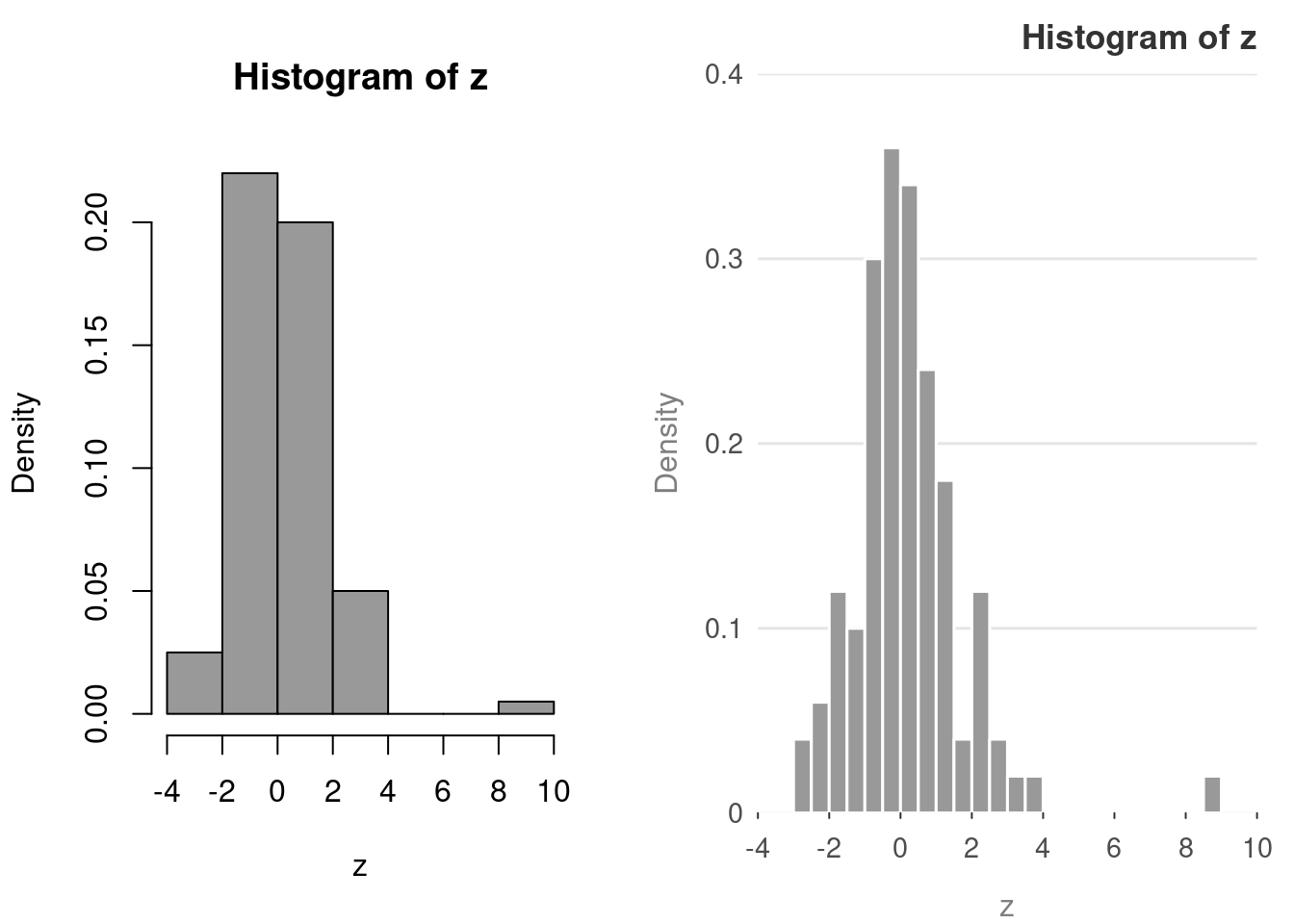## Barplot examples

The `barplot()` has an amazing number of different configurations.

``````op = par(mfrow = c(1, 2))
reset_prettyB()
library("grDevices") # for colours
tN = table(stats::rpois(100, lambda = 3))
barplot(tN)
barplot_p(tN)``````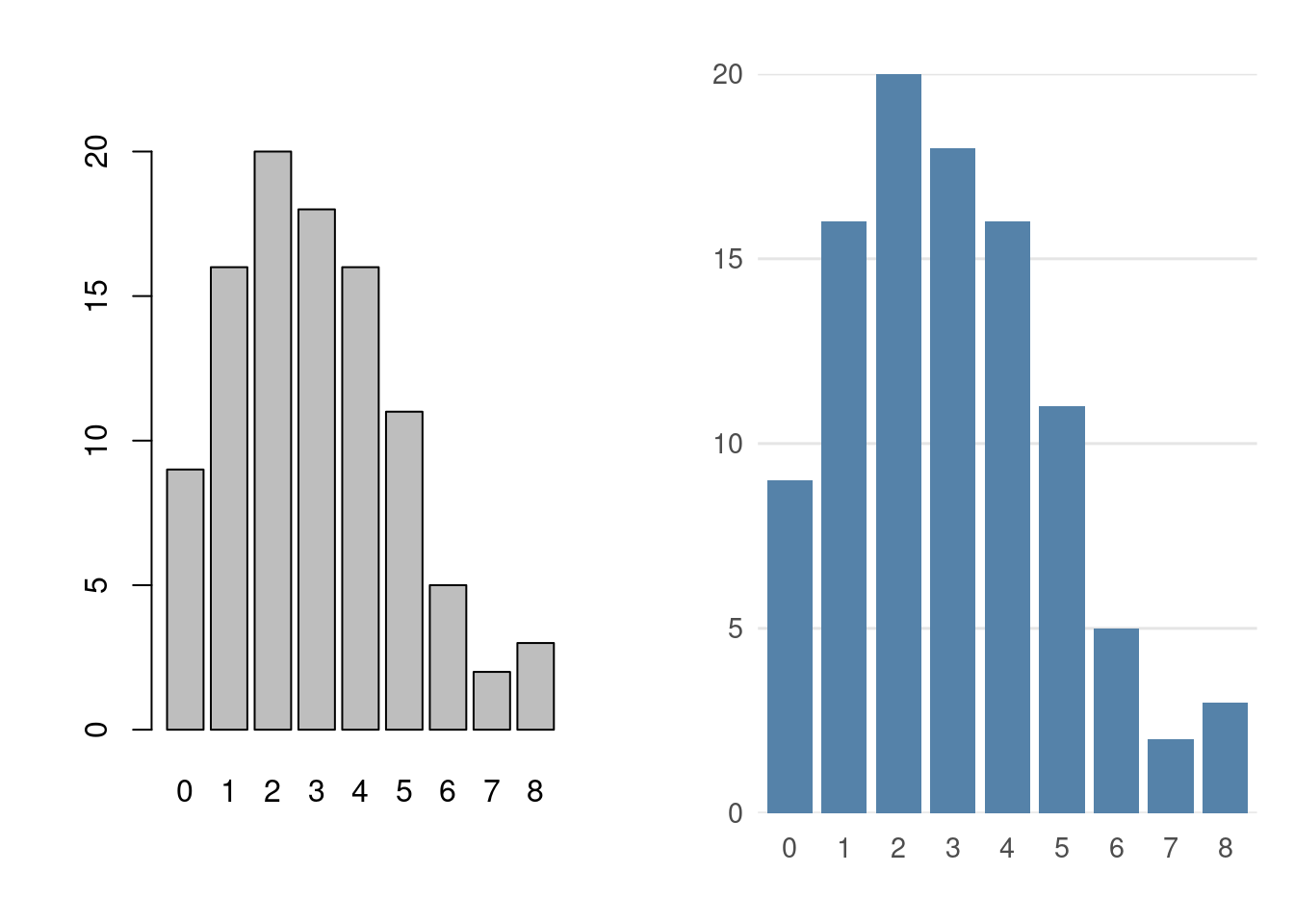The following examples are taken directly from the example page of `?barplot`. This means that sometimes the colours clash.

``````reset_prettyB()
r = barplot(tN, col = rainbow(20))
#- type = "h" plotting *is* 'bar'plot
lines(r, tN, type = "h", col = "red", lwd = 2)
r = barplot_p(tN, col = rainbow(20))
#- type = "h" plotting *is* 'bar'plot
lines(r, tN, type = "h", col = "red", lwd = 2)``````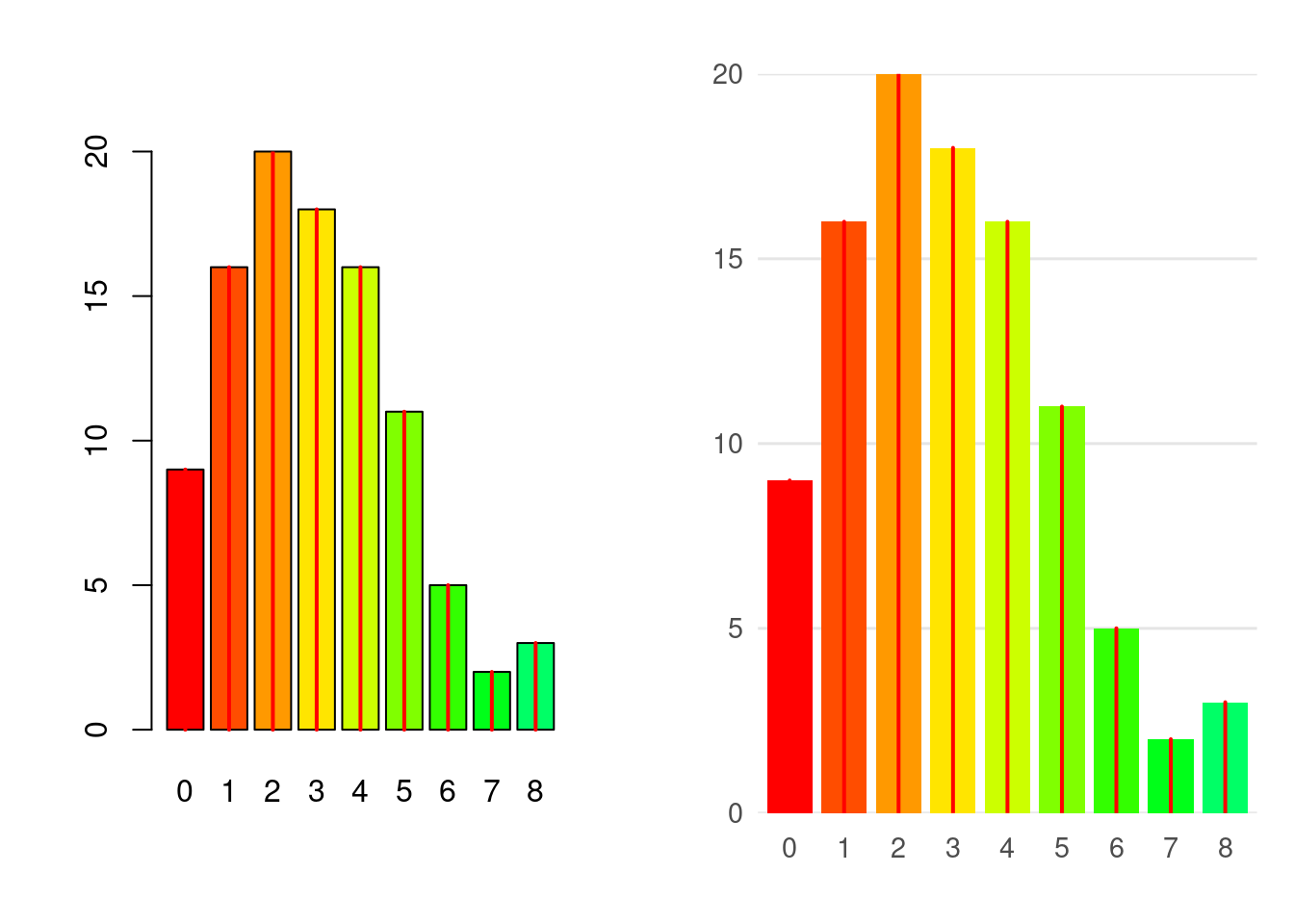Spacing and titles still work

``````reset_prettyB()
barplot(tN, space = 1.5, axisnames = FALSE,
sub = "barplot(..., space= 1.5, axisnames = FALSE)")
barplot_p(tN, space = 1.5, axisnames = FALSE,
sub = "barplot(..., space= 1.5, axisnames = FALSE)")``````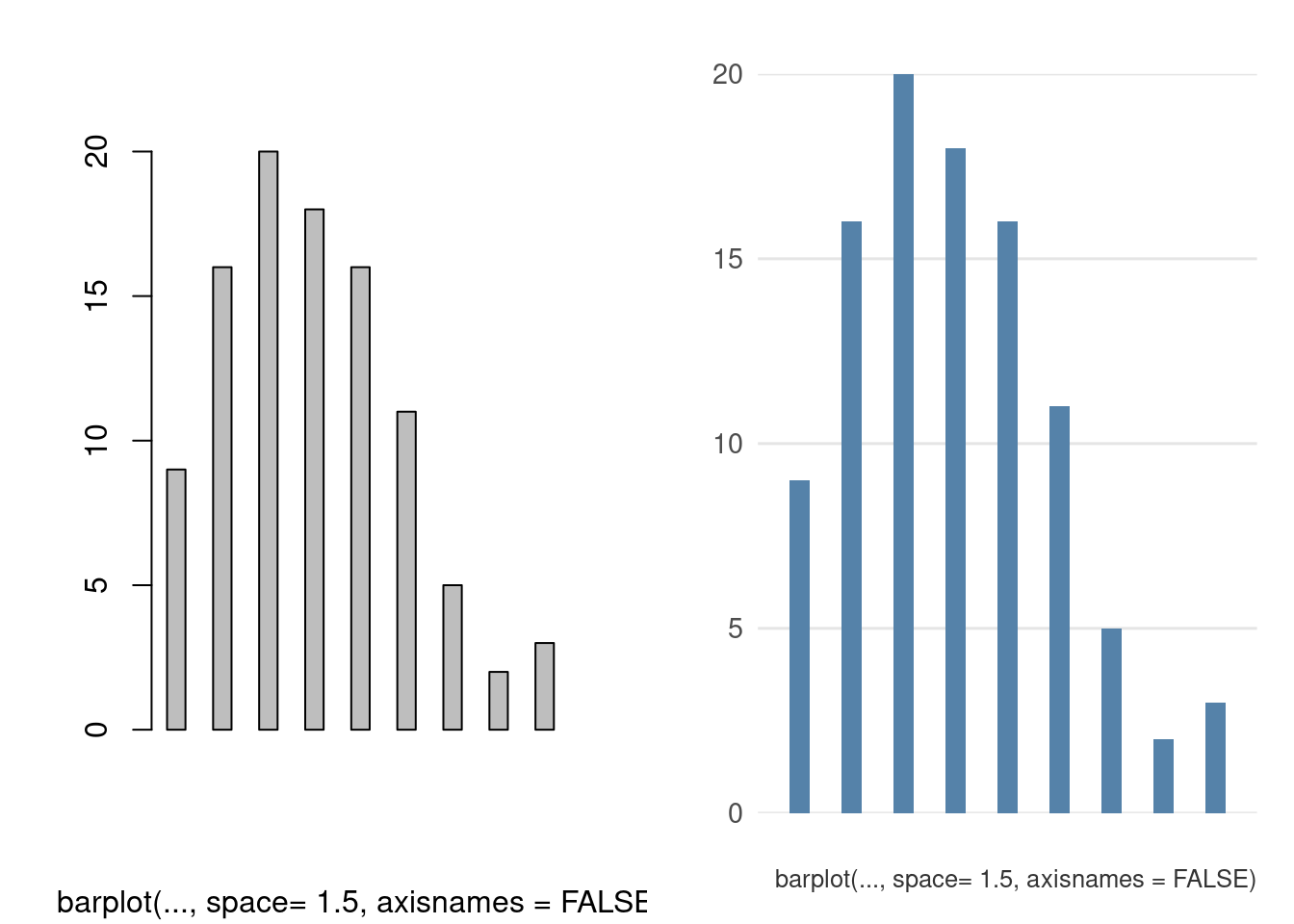So does the `beside` argument.

``````reset_prettyB()